# A membrane with voltage-dependent conductances increases the energy efficiency of fly photoreceptors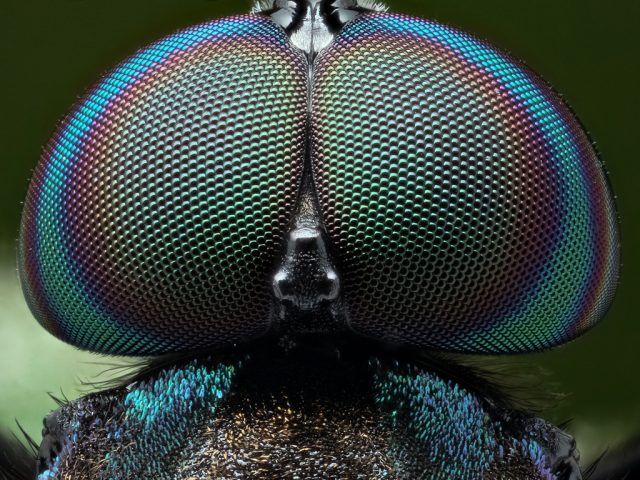The neuronal membrane, like membranes surrounding other cells, is mainly formed by a lipid surface impermeable to ions. It is only through ion channels, small protein pores embedded in the membrane, that ions can cross, allowing electrical current to charge or discharge the neuron. The inclusion of ion channels in a membrane, determines its electrical properties, with several consequences. For instance, it modifies the ability of the neuron to transmit information, either by producing action potentials or by transmitting graded potentials. At the same time, the ion channels modify the cost of keeping the neuron functioning and signalling.

A group of us, working in the Universities of Cambridge and Sussex (UK), published two articles with new insights about the role of ion channels in increasing the energy efficiency of fly photoreceptors, a well studied example of graded potential neuron. To be able to understand what we found, I need to explain first that the aggregate effect of many ion channels produces a conductance that is different to boring electrical resistors in several aspects. I will focus on two of them. First, ion channels (and thus the resulting conductance) are often selective, meaning that they let only certain ions through, and block ions of different types. Second, many ion channels are voltage-dependent, and either they open and let ions through, or they close and block them, depending on the history of voltage changes across the membrane.

Then, I need to introduce the simple and traditional, but strictu sensu wrong, way to relate the ability of a membrane to transmit fast signals to the photoreceptor energy consumption. The photoreceptor membrane was considered to behave as a simple RC circuit, whose capacitance is fixed because it depends on properties such as membrane area or thickness (difficult to change without compromising the photoreceptor performance). With a fixed capacitance, the bandwidth of an RC circuit is proportional to the conductance (Fig 1). Increasing the membrane conductance in a photoreceptor, however, means increasing ionic currents through the membrane. If signalling is to continue, the ionic currents must be reversed, an active process that is the major energy consumer in the photoreceptor. If the previous arguments are to be believed, the inescapable conclusion is that bandwidth is proportional to energy consumption, and thus it is impossible to increase one without increasing the other.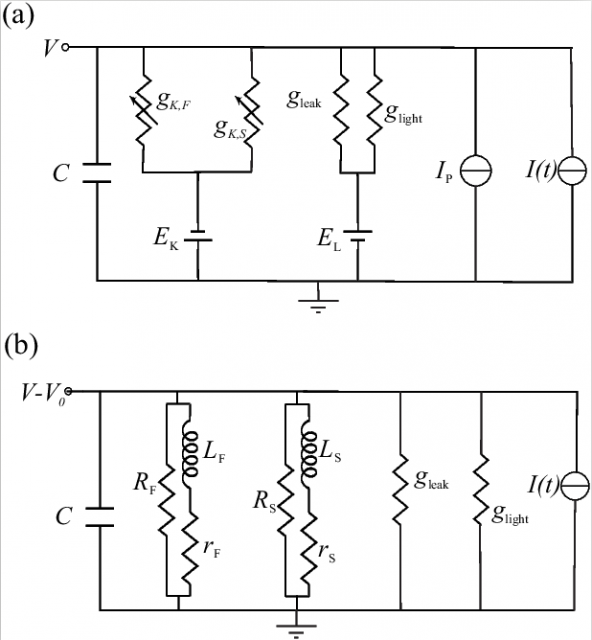Figure 2. Electric circuits of the blowfly membrane. (a) With voltage-dependent conductances (b) Whose voltage-dependent conductances have been substituted by the equivalent circuit of resistors and inductors reproducing small voltage deflections.

However, I have stated before that ion channels are not boring resistors. We discovered how two well-known properties of potassium ion channels allow the fly photoreceptor membrane to improve energy efficiency above what previous argument would allow, decreasing the energy needed to obtain a photoreceptor membrane of a given bandwidth. The first one is that certain ion channels behave electrically as a circuit containing inductors, electrical elements that respond with a voltage proportional to the change in current. Fly photoreceptor membranes contain the precise type of ion channels that introduce inductive effects (Figure 2). These inductive effects increase the membrane bandwidth, in the same way that inductive elements are sometimes added to amplifiers to increase their bandwidth, an strategy called shunt peaking. We show that in the blowfly photoreceptor, shunt peaking by potassium ion channels increases bandwidth by up to 50%, compared with what a boring resistor would do 1.

The second property of ion channels decreases the energy cost of membrane bandwidth by decoupling membrane resistance (the quantity that determines the flux of ions through the membrane, and thus energy cost), from input resistance and impedance (the magnitudes that relate the size of the voltage response to injected current). To see how, let us compare the electric responses to sustained current of a boring voltage-independent resistor, and a resistor whose resistance changes with the voltage between its ends. To simplify the operations, let us consider the conductance (the inverse of the resistance), which will be voltage-independent in the first case, and voltage-dependent in the second.

If we want to obtain a certain voltage drop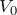between the ends of the boring resistor, we need to inject a constant current,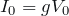. Any small increment or decrement in voltage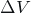, will require a change in current of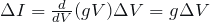. Both steady-state voltage and currents, and voltage and current increments, relate linearly through the same coefficient G.

Now consider a resistor that depends on voltage as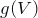. To cause a voltage drop ofwe need to inject a steady-state current of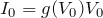, the same as above. If the voltage changes by a little amount, the voltage-dependent resistor reveals itself as a completely different animal, as it requires an increase in current of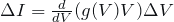=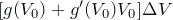. The coefficient relating steady current and voltage is now different to the one relating small changes in current to small changes in voltage. Varying how fast conductance changes with voltage around voltage, while keeping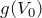constant, it is possible to change the responses to small current increments or decrements of current without changing the relation between steady-state current and voltage.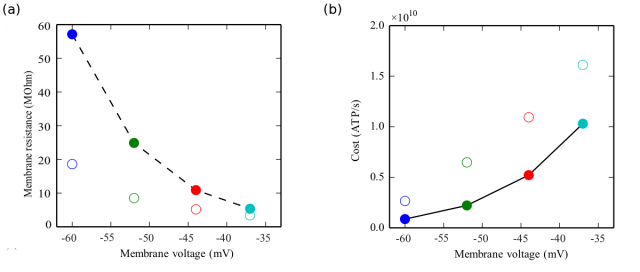Figure 3. Voltage-dependent conductances improve the energy efficiency of fly photoreceptors. To have a similar bandwidth, photoreceptor membranes with no voltage-dependent ion channels (empty circles) must have a lower resistance (a) an thus consume more energy (b) than photoreceptor with voltage-dependent ions (filled circles). This happens at different light levels, when the photoreceptor is depolarised to different membrane voltages.

This second effect of voltage-dependent conductances, lowering impedance and input resistance without lowering conductance, was already known, but we were the first to identify it as an energy-saving strategy 2. To see the net effect of both shunt-peaking and the decoupling of filtering and resistance in the energy cost of the blowfly photoreceptor membrane bandwidth, we compared an “active” model of the photoreceptor membrane, with voltage-dependent conductances, to “passive” membrane models that obtain the same bandwidth by using voltage-independent conductances. As we expected, the passive membranes had to decrease their membrane resistance compared to the active photoreceptor membrane (Figure 3a). This decrease in membrane resistance in the passive membrane involves an increase in ionic current, and thus an increase in the energy expended in restoring the ionic concentration inside and outside the cell (Figure 3b). Therefore, through the use of voltage-dependent potassium ion channels, the blowfly photoreceptor saves between 30% and 60% compared to passive membranes with only voltage-independent conductances.

Our two articles answer some questions, but also open new ones. Potassium ion channels in the photoreceptor have properties that vary across different species of fly, correlating with their ecological niche. How do function and constraints determine that potassium ion channel properties must be different in different species? Do the potassium ion channels in other fly photoreceptors also decrease the energy cost of bandwidth? Also, as voltage-dependent ion channels are widespread in graded potential neurons, and in the dendrites of spiking neurons, the two strategies might be used to save energy in lots of neurons. Maybe our own brain use them to help energy efficiency?

## References

1. FJH Heras, SB Laughlin, JE Niven, “Shunt peaking in neural membranes”. Journal of The Royal Society Interface 13 (124), 20160719 doi:
2. FJH Heras, J Anderson, SB Laughlin, JE Niven, “Voltage-dependent K+ Channels Improve the Energy Efficiency of Signalling in Blowfly Photoreceptors”. Journal of The Royal Society Interface 14 (129), 20160938 doi: